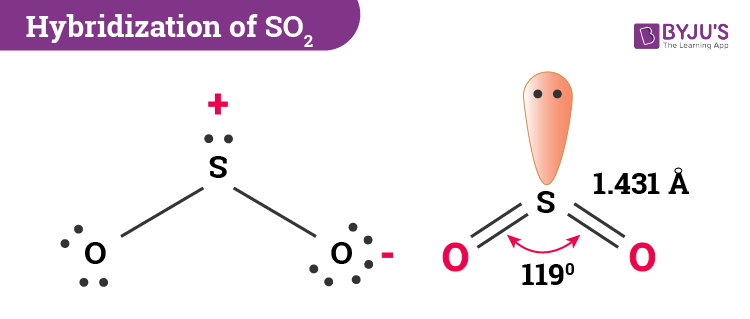Checkout JEE MAINS 2022 Question Paper Analysis : Checkout JEE MAINS 2022 Question Paper Analysis :

# Hybridization of SO2 (Sulphur Dioxide)

Here, we will explain the hybridization of SO2 in detail. Students will also learn how to determine the type of hybridization, and they will find information about SO2 molecular geometry and bond angles.

 Name of the Molecule Sulphur Dioxide Molecular Formula SO2 Hybridization Type sp2 Bond Angle 119o Geometry V-Shaped or Bent

## What is the Hybridization of Sulphur Dioxide?

In sulphur dioxide, the hybridization that takes place is sp2 type. To determine this, we will first look at the sulphur atom which will be the central atom. During the formation of SO2, this central atom is bonded with two oxygen atoms and their structure can be represented as O=S=O. As for the bonding, there is one sigma and one pi bond formed between sulphur and the two oxygen atoms. The atom will also accommodate one lone pair.Let us break it down further. Looking at the ground state of sulphur consists of six electrons in the outermost shell and the first two shells are also completely filled. There are 4 electrons in the 3p portal and two paired electrons in the 3s orbital. Now, it has to form four bonds (with oxygen) and thus needs 4 unpaired electrons. This leads to the development of the excited state in sulphur where one 3px electron jumps to the 3d orbital. When this happens there will be one unpaired electron in one 3d orbital and three in 3p orbitals. However, the electrons that form the sigma bonds and the lone pair are at different energy levels. The stable state is obtained when hybridization takes place.

During hybridization, two 3p orbitals and one 3s orbital get hybridized. In total, there is a formation of three sp2 hybrid orbitals. In this, two hybrid orbitals contain unpaired electrons and one hybrid orbital will have the lone pair. These unpaired electrons then form sigma bonds with the oxygen atoms. Meanwhile, the 3d and 3p orbitals remain the same, and they take part in the formation of pi bonds.

Interestingly, the hybridization of the oxygen atom in this compound is also sp2.

### Important Points To Remember

• In SO2 hybridization two 3p orbitals and one 3s orbital get hybridized.
• Two-hybrid orbitals contain unpaired electrons and one hybrid orbital will have the lone pair.
• The 3d and 3p orbitals remain the same, and they form pi bonds.

## SO2 Molecular Geometry And Bond Angles

SO2 molecular geometry is considered to V-shaped or bent. Alternatively, the electron geometry of sulphur dioxide is in the shape of a trigonal planar. The three pairs of bonding electrons lie at an angle of 119o. Two double pairs are bonded together and there is one lone pair as well which further gives it a bent shape.

## Chemical Bonding## Frequently Asked Questions – FAQs

### What is the hybridization, shape and geometry of SO2?

SO2 is sp2 hybridized with trigonal planar geometry and has a bent shape.

### What is the bond angle of the sulfur dioxide molecule?

The bond angle of the sulfur dioxide molecule is 119°.

### Which orbitals are involved in the hybridization of sulfur dioxide?

The 3p and 3s orbital is involved in the hybridization of sulfur dioxide.

### What bonds are present in the sulfur dioxide molecule?

Sulfur dioxide molecule contains sigma and pi bonds.
Test Your Knowledge On Hybridization Of So2!

#### 1 Comment

1. ROHAN ROY

thanks its helping us a lot